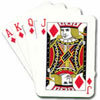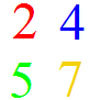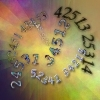Search by Topic

Resources tagged with Permutations similar to Six Notes All Nice Ratios:

Filter by: Content type:
Age range:
Challenge level:

There are 17 results

Broad Topics > Decision Mathematics and Combinatorics > PermutationsFactoring a Million

Age 14 to 16 Challenge Level:

In how many ways can the number 1 000 000 be expressed as the product of three positive integers?Thank Your Lucky Stars

Age 14 to 16 Challenge Level:

A counter is placed in the bottom right hand corner of a grid. You toss a coin and move the star according to the following rules: ... What is the probability that you end up in the top left-hand. . . .Even Up

Age 11 to 14 Challenge Level:

Consider all of the five digit numbers which we can form using only the digits 2, 4, 6 and 8. If these numbers are arranged in ascending order, what is the 512th number?Six Times Five

Age 11 to 14 Challenge Level:

How many six digit numbers are there which DO NOT contain a 5?Master Minding

Age 11 to 14 Challenge Level:

Your partner chooses two beads and places them side by side behind a screen. What is the minimum number of guesses you would need to be sure of guessing the two beads and their positions?Painting Cubes

Age 11 to 14 Challenge Level:

Imagine you have six different colours of paint. You paint a cube using a different colour for each of the six faces. How many different cubes can be painted using the same set of six colours?Ding Dong Bell

Age 11 to 18

The reader is invited to investigate changes (or permutations) in the ringing of church bells, illustrated by braid diagrams showing the order in which the bells are rung.Card Shuffle

Age 11 to 16

This article for students and teachers tries to think about how long would it take someone to create every possible shuffle of a pack of cards, with surprising results.396

Age 14 to 16 Challenge Level:

The four digits 5, 6, 7 and 8 are put at random in the spaces of the number : 3 _ 1 _ 4 _ 0 _ 9 2 Calculate the probability that the answer will be a multiple of 396.And So on and So On

Age 11 to 14 Challenge Level:

If you wrote all the possible four digit numbers made by using each of the digits 2, 4, 5, 7 once, what would they add up to?Euromaths

Age 11 to 14 Challenge Level:

How many ways can you write the word EUROMATHS by starting at the top left hand corner and taking the next letter by stepping one step down or one step to the right in a 5x5 array?Permute It

Age 11 to 14 Challenge Level:

Take the numbers 1, 2, 3, 4 and 5 and imagine them written down in every possible order to give 5 digit numbers. Find the sum of the resulting numbers.Bell Ringing

Age 11 to 14 Challenge Level:

Suppose you are a bellringer. Can you find the changes so that, starting and ending with a round, all the 24 possible permutations are rung once each and only once?Age 14 to 18 Challenge Level:

Some relationships are transitive, such as `if A>B and B>C then it follows that A>C', but some are not. In a voting system, if A beats B and B beats C should we expect A to beat C?Flagging

Age 11 to 14 Challenge Level:

How many tricolour flags are possible with 5 available colours such that two adjacent stripes must NOT be the same colour. What about 256 colours?Power Crazy

Age 11 to 14 Challenge Level:

What can you say about the values of n that make $7^n + 3^n$ a multiple of 10? Are there other pairs of integers between 1 and 10 which have similar properties?Chances Are

Age 14 to 16 Challenge Level:

Which of these games would you play to give yourself the best possible chance of winning a prize?